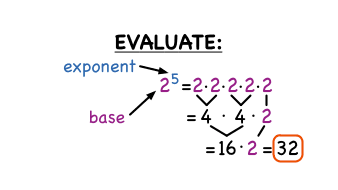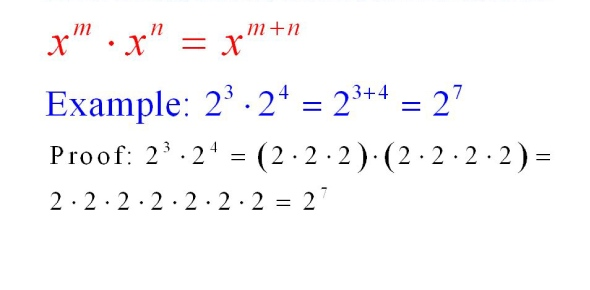~What Is An Exponent?

An exponent is how much times a number is being multiplied by itself. In other words how many copies of itself it’s making. For example if a have a number like 2 to the power of 3, in this case the 2 is being copied 3 times. This is equal to 8. Another example of an exponent is a power. A power is basically what an exponent is but using a fancier word. I will get into more of what you can do with an exponent and just how important they are in math.~What Is The Difference Between Evaluating and Simplifying?

The difference of evaluating and simplifying is quite simple. Evaluating means actually doing the equation and figuring out what for example 5 to the power of 2. Simplifying is subtracting exponents using three laws. The first one is the multiplication law, then the division law and lastly the power law. For example if I have 5 to the power of 6 multiplied by 5 to the power of 3 in this case we add the exponents so the answer would be 5 to the power of 9. Another example is using the division law which is subtraction. If we have 4 to the power of 7 subtracted by 4 to the power of 5 since we only subtract the exponents the answer becomes 4 to the power of 2. I will explain each of these laws more thoroughly and how they work.~Multiplication Law and How It Works

What is the multiplication law? The multiplication law is where if you have two numbers that have the same base and is a multiplication question, you can leave the bases the way they are and add the exponents. For example, if I have 6 to the power of 2 multiplied by 6 to the power of 8, since the bases are the same we are able to add the exponents  so therefore the answer is 6 to the power of 10. Well what if we have a negative base multiplied by a negative base? That’s a good question what happens is for example if we have (-6) to the power of 3 multiplied by (-6) to the power of 2 as I said before the bases aren’t the things that are changing its the exponents. Since we know that we add the exponents the answer becomes (-6) to the power of 5. That’s how the multiplication law works with both positive and negative numbers.~Division Law and How It Works

What is the division law? The division law is the same concept as the multiplication law but instead of adding the exponents, you subtract them. For example if we have 4 to the power of 6 divided by 4 to the power or 3 we subtract the exponents and up with 4 to the power of 3. What is it’s a negative? Just like the multiplication law the bases aren’t the things that are changing but its the exponents that are. So if we have (-5) to the power of 7 divided by (-5) to the power of 5 we subtract 7 from 5 and you get (-5) to the power of 2. Oh wait we forgot something, since the exponent is even the base becomes positive therefore our new and final answer is 5 to the power of 2. The picture on the very left is an example of the first example I mentioned and since 4 divided by 4 is one we are doing it 3 times and we are left with three four’s left over.  Therefore our answer is 4 to the power of 3. Just like the picture on the left, the picture on the right is using the same example but only negatives and it also shows that when you have a even exponent with a negative base it becomes a positive base. That’s how the division law works.

~The Power Law and How It Works

Out of the three laws this law uses 2 exponents and brackets in an equation. For example if we have (2 to the power of 3) and outside of the bracket we a have a 2 that’s an example of a power multiplied by a power or the power law. So in this case we leave the base and multiply the exponents. Therefore the answer is 2 to the power of 6. What if the base is negative? The thing about these three laws is that they are connected in at least one or two ways and this is where it connects. YOU NEVER CHANGE THE BASE ONLY THE EXPONENTS. So if we have (-2 to the power of 3) and a 2 outside the answer is (-2) to the power of 6. Again it has an even exponent so our final answer is 2 to the power of 6.~Applications of Exponents

An application of exponents is Pythagoras. Pythagoras is a squared multiplied by b squared equals c squared. For example if we have a question with a right triangle like the one below that says 5 one side and 7 on the bottom side and c on the longest side, we know that this is a pythagoras question. We can immediately identify the hypotenuse is the because it’s the longest side of the triangle. Our first step is to square the 5 and 7 so we end up getting 25 and 49. Now the second step is to add the two so we get 74. Now we have our C. There is one more step. We have to find the square root of 74. In this case it’s 8.6 and that’s our final answer. That’s an application of exponents.~One More Thing I Learned About Exponents

One more thing I learned about exponents is that there is a thing called a coefficient. A coefficient is basically an invisible 1 when a whole number is by itself because it doesn’t matter so you leave a whole number as is but there always is one. When working with exponents it’s very important because when you are simplifying equations and there is just a whole number, there is actually an invisible 1 that you have to add as well so it’s really important to keep coefficients in mind. This also applies when an exponent is a 0. For example if we have 2 to the power of 0 since anything multiplied by 0 becomes 0, in this case there is a coefficient so it becomes 1. This is very important so I am happy that I was able to learn what a coefficient was.~Scources

http://www.solving-math-problems.com/math-exponents.html

https://www.proprofs.com/discuss/q/340683/the-multiplication-law-exponents-says-that-for-any-numbers-a

https://virtualnerd.com/middle-math/number-algebraic-sense/powers-exponents/evaluate-exponents-examples